# 3. Java 基本结构 ​

## 3.1 一个简单的程序 ​

``````[package 包名]
impoort 包名.类名
[public] class 类名 {
int val = '123';
public static void main(String args[]) {
// 变量定义和语句
}
}``````
java
``````public class WelcomeSimple
{
public static void main(String[] args)
{
String greeting = "Hello world";
System.out.println(greeting);
}
}``````
• Java 区分大小写
• 文件名和类名相同
• 必须包含 - `main` - 方法
• main 方法必须是 public 修饰符

## 3.2 注释 ​

java
``````// single line comment

/*
more
line
comment
*/

/**
* document
* more
* line
* comment
*/``````

## 3.3 数据类型 ​

### 3.3.1 整形 ​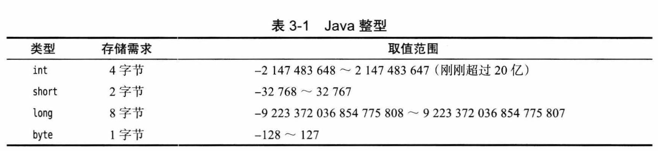``````long intLong = 400000000l;
int intHex = 0x1654AC;
short intOct = 010;
int intBin = 0b10001;

### 3.3.2 浮点类型 ​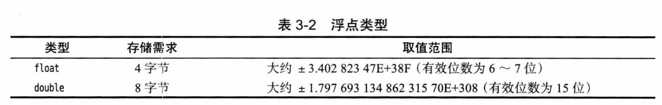``````float floatFloat = 3.14f;
double floatDouble = 3.14d;``````

``````Double.POSTTIVE_INFINITY  // 正无穷
Double.NEGATIVE_INFTNIIY  // 负无穷
Double.NaN                // 不是数值``````

### 3.3.3 Char ​

`\u0000 - \uFFFF` 的 16 进制的数据, 这里的特殊情况需要注意

``"\u0022+\u0022" 并不是一个由引号包围加号构成的字符串, 因为 \u0022 会转换为引号会得到 ""+"" 也就是空字符串``

``````// \u000A is a newline    :  \u000A 会转换为换行, 这里就会产生语法错误
// look inside c:\users   :  \users 不是一个有效的 16 进制数, 会报语法错误``````

### 3.3.4 unicode 和 char 类型 ​

unicode 官方网站 : https://home.unicode.org/

utf-8 是 unicode 编码的一种实现方式

https://www.ruanyifeng.com/blog/2007/10/ascii_unicode_and_utf-8.html

true/false

## 3.4 变量与常量 ​

### 3.4.1 声明变量 ​

``int score;``

### 3.4.2 变量初始化 ​

``````int days;
days = 12;

int days = 12;``````

``var days = 28;``

### 3.4.2 常量 ​

java
``````public class Constants
{
public static final double CMPERINCH = 2.54;   // 类常量

public static void main(String[] args)
final double MC_PER_INCH = 2.54;           // 常量
double paperwidth = 8.5;
}
}``````

### 3.4.3 枚举 ​

``enum Size { SMALL, MEDIUM, LARGE, EXTRA_LARGE }``

## 3.5 运算符 ​

### 3.5.1 算术运算符 ​

 操作符 描述 例子 + 加法 - 相加运算符两侧的值 A + B等于30 - 减法 - 左操作数减去右操作数 A – B等于-10 * 乘法 - 相乘操作符两侧的值 A * B等于200 / 除法 - 左操作数除以右操作数 B / A等于2 ％ 取模 - 左操作数除右操作数的余数 B%A等于0 ++ 自增: 操作数的值增加1 B++ 等于21 – 自减: 操作数的值减少1 B– 等于19

### 3.5.2 数学函数与常量 ​

``double y = Math.sqrt(x);``

### 3.5.3 数值之间的转换 ​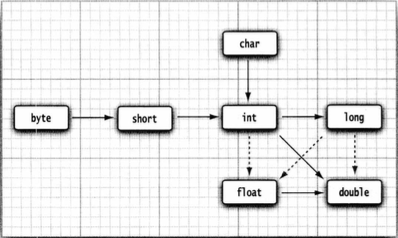### 3.5.4 强类型转换 ​

``````double x = 9.97;
int nx = (int) x;   // 9``````

### 3.5.5 结合赋值和运算符 ​

``````× += 4 ;
x = x + 4;``````

### 3.5.6 自增与自减运算符 ​

``````n++;
n--;``````

### 3.5.7 关系和 boolean 运算符 ​

 运算符 描述 例子 `==` 检查如果两个操作数的值是否相等，如果相等则条件为真。 （A == B）为假(非真)。 `!=` 检查如果两个操作数的值是否相等，如果值不相等则条件为真。 (A != B) 为真。 `>` 检查左操作数的值是否大于右操作数的值，如果是那么条件为真。 （A> B）非真。 `=` 检查左操作数的值是否大于或等于右操作数的值，如果是那么条件为真。 （A> = B）为假。 `<=` 检查左操作数的值是否小于或等于右操作数的值，如果是那么条件为真。 （A <= B）为真。

### 3.5.8 位运算符 ​

 操作符 描述 例子 `＆` 如果相对应位都是1，则结果为1，否则为0 （A＆B），得到12，即0000 1100 `^` 如果相对应位值相同，则结果为0，否则为1 （A ^ B）得到49，即 0011 0001 `〜` 按位补运算符翻转操作数的每一位，即0变成1，1变成0。 （〜A）得到-61，即1100 0011 `>` 按位右移运算符。左操作数按位右移右操作数指定的位数。 A >> 2得到15即 1111 `>>>` 按位右移补零操作符。左操作数的值按右操作数指定的位数右移，移动得到的空位以零填充。 A>>>2得到15即0000 1111

### 3.5.9 括号与运算符级别 ​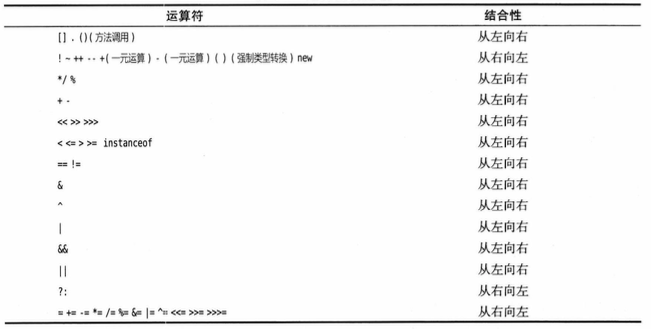## 3.6 字符串 ​

### 3.6.1 子串 ​

``````String greeting = "Hello";
String s = greeting.substring(0，3);``````

### 3.6.2 拼接 ​

``````String greeting = "Hello";
String guide = greeting + "World."``````

### 3.6.5 空和 null 串 ​

``````str.length() == 0

str.equals(""))``````

``str == null``

``str != null && str.length() != 0``

### 3.6.8 查看文档 ​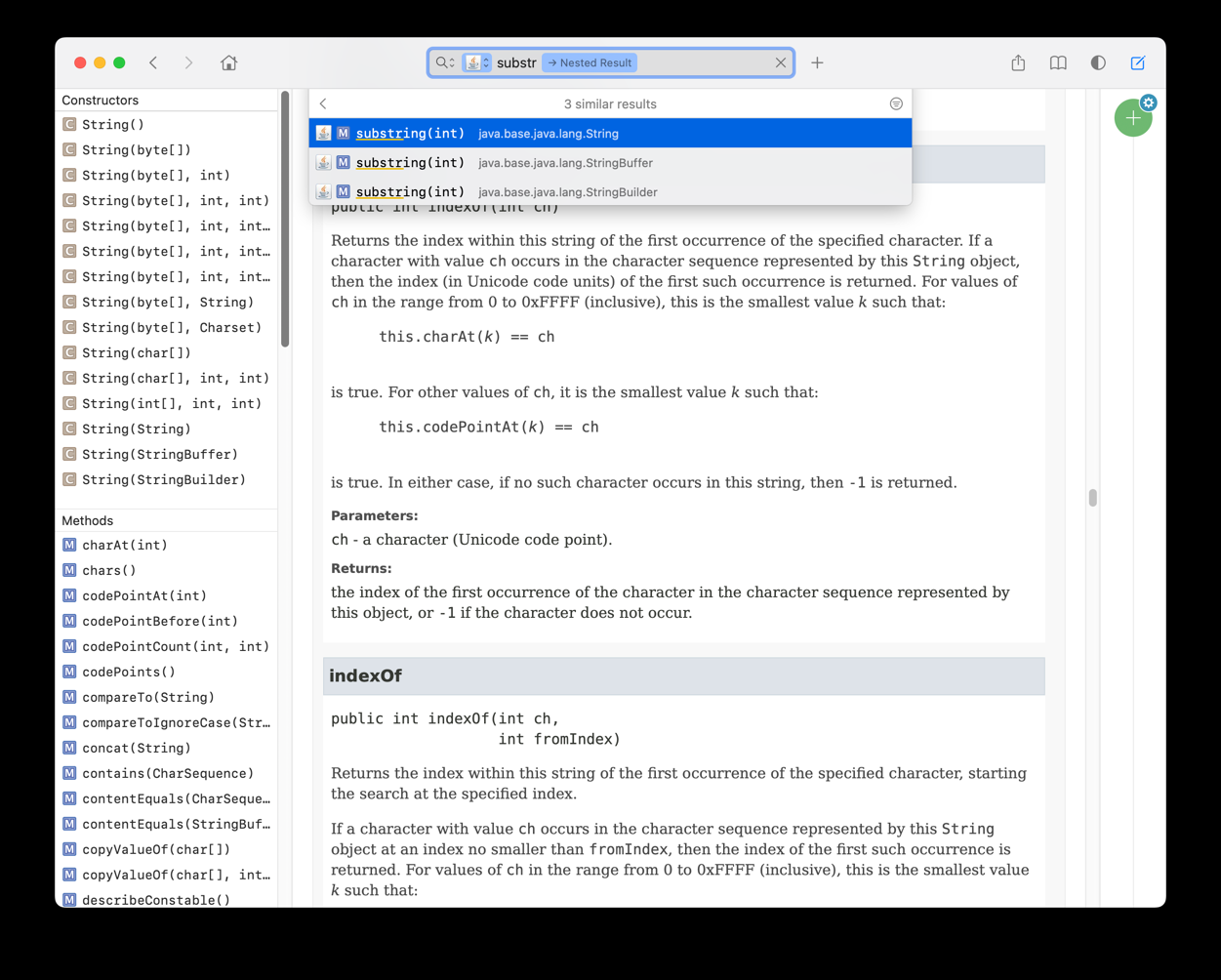### 3.6.9 构建字符串 ​

java
``````StringBuilder builder = newStringBuilder();
builder.append(ch);  // appends a single character
builder.append(str); // appends a string
StringcompletedString = builder.toString() ;``````

### 3.6.10 字串的转义 ​

``````\n       换行 (0x0a)
\r       回车 (0x0d)
\f       换页符(0x0c)
\b       退格 (0x08)
\s       空格 (0x20)
\t       制表符
\"       双引号
\'       单引号
\\       反斜杠
\ddd     八进制字符 (ddd)
\uxxxx   16进制Unicode字符 (xxxx)``````